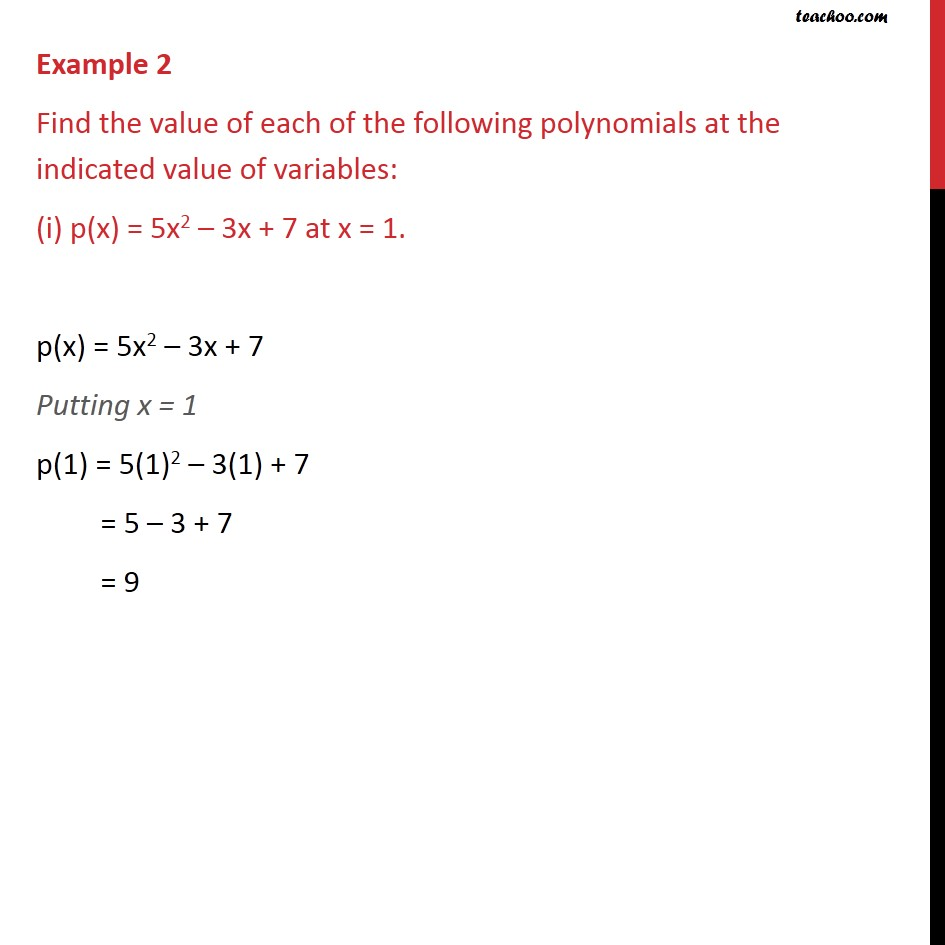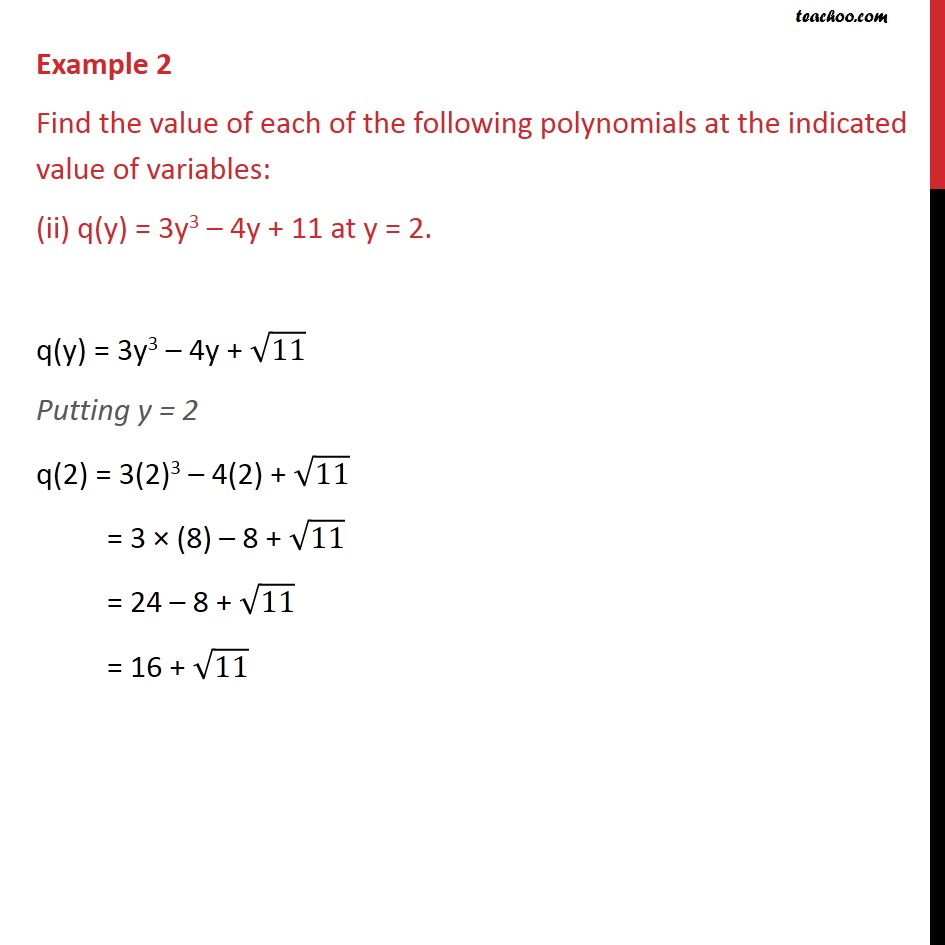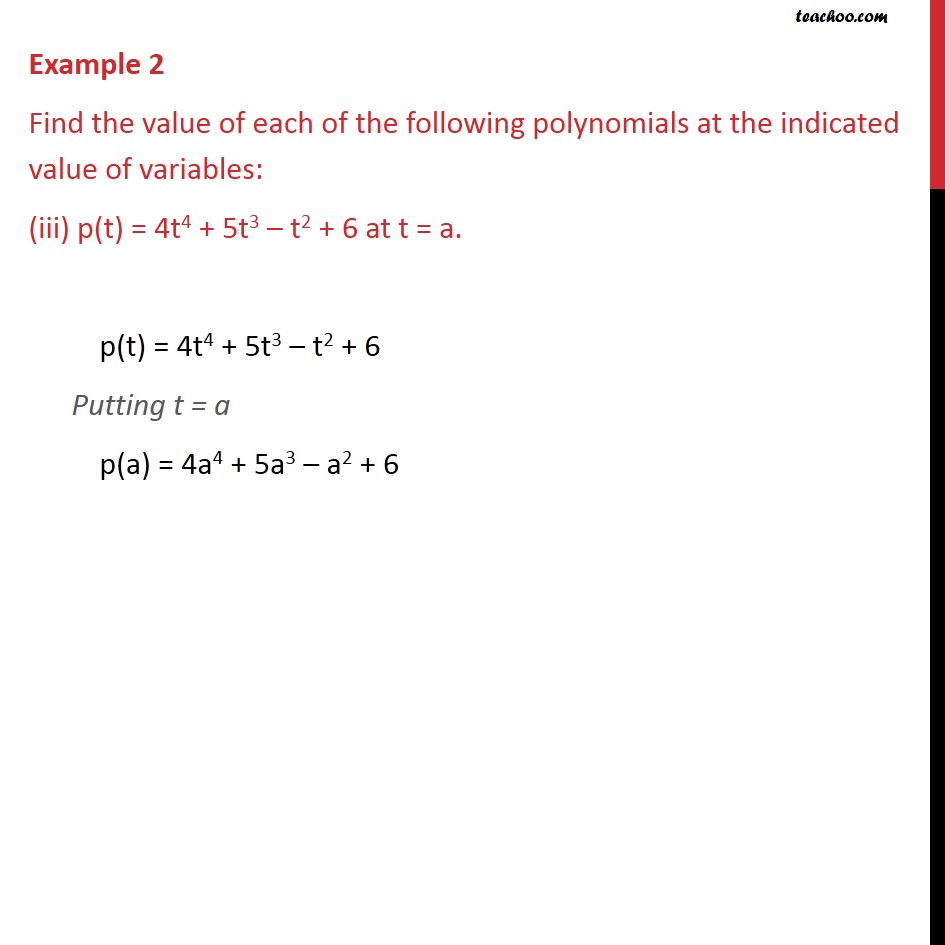Learn all Concepts of Polynomials Class 9 (with VIDEOS). Check - Polynomials Class 91. Chapter 2 Class 9 Polynomials
2. Concept wise
3. Value of a polynomial at a given point

Transcript

Example 2 Find the value of each of the following polynomials at the indicated value of variables: (i) p(x) = 5x2 – 3x + 7 at x = 1. p(x) = 5x2 – 3x + 7 Putting x = 1 p(1) = 5(1)2 – 3(1) + 7 = 5 – 3 + 7 = 9 Example 2 Find the value of each of the following polynomials at the indicated value of variables: (ii) q(y) = 3y3 – 4y + 11 at y = 2. q(y) = 3y3 – 4y + √11 Putting y = 2 q(2) = 3(2)3 – 4(2) + √11 = 3 × (8) – 8 + √11 = 24 – 8 + √11 = 16 + √11 Example 2 Find the value of each of the following polynomials at the indicated value of variables: (iii) p(t) = 4t4 + 5t3 – t2 + 6 at t = a. p(t) = 4t4 + 5t3 – t2 + 6 Putting t = a p(a) = 4a4 + 5a3 – a2 + 6

Value of a polynomial at a given point

About the AuthorDavneet Singh
Davneet Singh is a graduate from Indian Institute of Technology, Kanpur. He has been teaching from the past 9 years. He provides courses for Maths and Science at Teachoo.